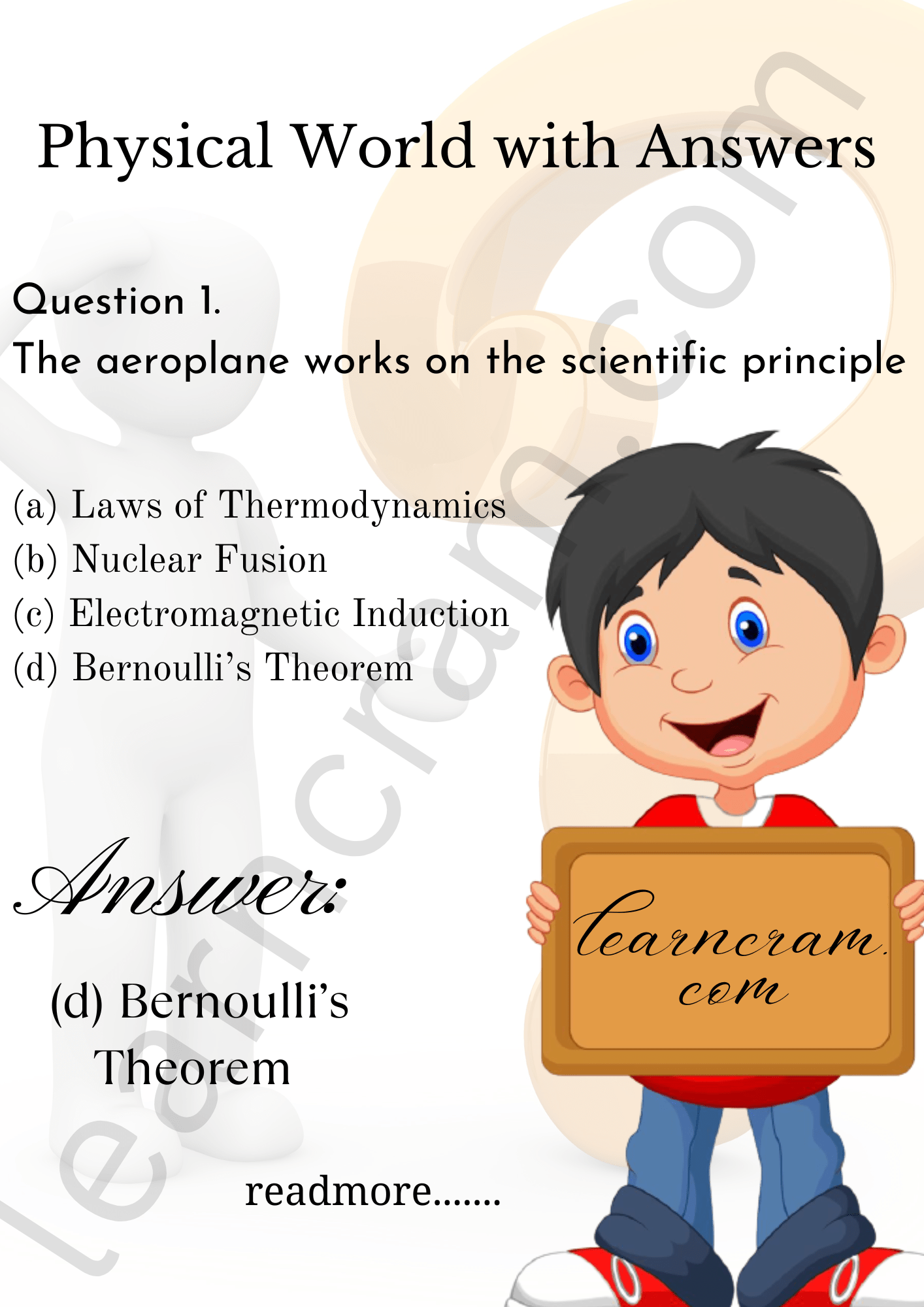# MCQ Questions for Class 11 Physics Chapter 1 Physical World with Answers

We have compiled the NCERT MCQ Questions for Class 11 Physics Chapter 1 Physical World with Answers Pdf free download covering the entire syllabus. Practice MCQ Questions for Class 11 Physics with Answers on a daily basis and score well in exams. Refer to the Physical World Class 11 MCQs Questions with Answers here along with a detailed explanation.

## Physical World Class 11 MCQs Questions with Answers

Multiple Choice Type

Question 1.
The aeroplane works on the scientific principle
(a) Laws of Thermodynamics
(b) Nuclear Fusion
(c) Electromagnetic Induction
(d) Bernoulli’s TheoremQuestion 2.
Nuclear reactor works on the scientific principle
(a) Bernoulli’s Theorem
(b) Nuclear Fission
(c) Electromagnetic Induction
(d) Laws of ThermodynamicsQuestion 3.
Who discovered neutron
(a) Galileo
(c) C.V. Raman
(d) None of them

Question 4.
Wave nature of electron was discovered by
(a) Roentgen
(b) Pauli
(c) de Broglie
(d) Einstein

Question 5.
Atomicity of charge was discovered by
(b) Marie Curie
(c) Maxwell
(d) R.A. Millikan

Question 6.
Theory of relativity was discovered by
(a) Maxwell
(c) Einstein
(d) Pauli

Question 7.
Electric generator works on the scientific principle
(a) Laws of Thermodynamics
(b) Nuclear Fusion
(c) Bernoulli’s Theorem
(d) Electromagnetic Induction

Question 8.
Size of the nucleus is
(a) 10-10 m
(b) 10-14 m
(c) 10-9 m
(d) 10-12 m

Question 9.
The scientists wh” discovered natural radioactivity belonged to
(a) Italy
(b) Austria
(c) France
(d) England

Question 10.
The scientist who discovered x-rays belonged to:
(a) Germany
(b) Japan
(c) England
(d) France

Question 11.
The scattering of light by molecules was discovered by
(a) J. Bardeen
(b) Einstein
(c) C.V. Raman
(d) Newton

Question 12.
The scientist who discovered the “Theory of Relativity” belonged to
(a) India
(b) France
(c) Germany
(d) None of these

Question 13.
Which of the following technology uses Newton’s second and third laws of motion
(a) Electric Generator
(c) Rocket Propulsion
(d) None

Question 14.
Laws of electromagnetic induction were discovered by :
(b) Einstein
(c) Newton

Question 15.
Heat engine and refrigerator work on scientific principle :
(a) Electromagnetic Induction
(b) Bernoulli’s Theorem
(c) Nuclear Fusion
(d) Laws of Thermodynamics

Question 16.
Who discovered cosmic rays
(a) J.J. Thomson
(b) Hess
(c) Lawrence
(d) Yukawa

Question 17.
The scientist who discovered cosmic rays belonged to
(a) America
(b) Austria
(c) India
(d) France

Question 18.
The study of the biological specimens are conducted at:
(a) Atomic level
(b) Ionic level
(c) Molecular level
(d) None

Question 19.
The physical sciences deal with the study of:
(a) Living things
(b) Non-living things
(c) Both
(d) None

Question 20.
The branch of science which deals with the study of stars is called
(a) Astronomy
(b) Geology
(c) Meterology
(d) Seismology

Fill in the blanks:

Question 1.
……………… was discovered by Huygen.

Question 2.
……………… was discovered by Hubble.

Question 3.
……………… is always attractive while ……………… force can be attractive or repulsive.

Question 4.
Matter is mostly electrically ……………… thus the electric force is largely ……………… and the gravitational force ……………… terrestrial phenomena.

Question 5.
Unification of weak and electromagnetic interactions was discovered by ………………

Question 6.
Abdus Salam belongs to ………………

Question 7.
The weak force are ……………… times stronger than the gravitational forces.

Question 8.
The electromagnetic force are ……………… times stronger than the gravitational forces and ……………… stronger than the weak forces.

Question 9.
The π-meson is the fieid particle of ……………… force while ……………… is the field particle of electromagnetic force.

Question 10.
……………… is the long range force while ……………… is the short range force.

Question 11.
……………… is a central as well as a conservative force.

True/False Type Questions

Question 1.
T.V. is based on the laws of thermodynamics and heat engine on electromagnetic waves.

Question 2.
Digital logic is the basis for Computer and Third law of motion is the principle of rocket launching.

Question 3.
The nuclear reaction is the basis of nuclear reactor and laws of thermodynamics are the basis of thermal power plant.

Question 4.
Newton’s laws of motion are used in laser and light amplification in rocket propulsion.

Question 5.
A. Einstein received noble prize for E = mc² and Raman for hydrogen spectra.

Question 6.
Biological sciences deal with the study of living things.

Question 7.
Telephone was discovered by Grahm Bel.

Question 8.
Electric bulb and telegraphy were discovered by Edison.

Question 9.
Wave theory of light was discovered by de-Broglie.

Question 10.
Theory of nuclear forces was discovered by H. Yukawa.

Match Type Questions

Match the scientist’s name against his discovery.

 Scientist Discovery Faraday (a) Inelastic scattering of light by molecules Bohr (b) Law of gravitation Rutherford (c) Atomic theory Chandwick (d) Expansion of universe Maxwell (e) Theory of relativity Einstein (f) Unification of light and electromagnetism Hubble (g) Discovery of neutron Dirac (h) Nuclear model of atom Newton (i) Quantum Theory of hydrogen atom Raman (j) Laws of electromagnetic Induction